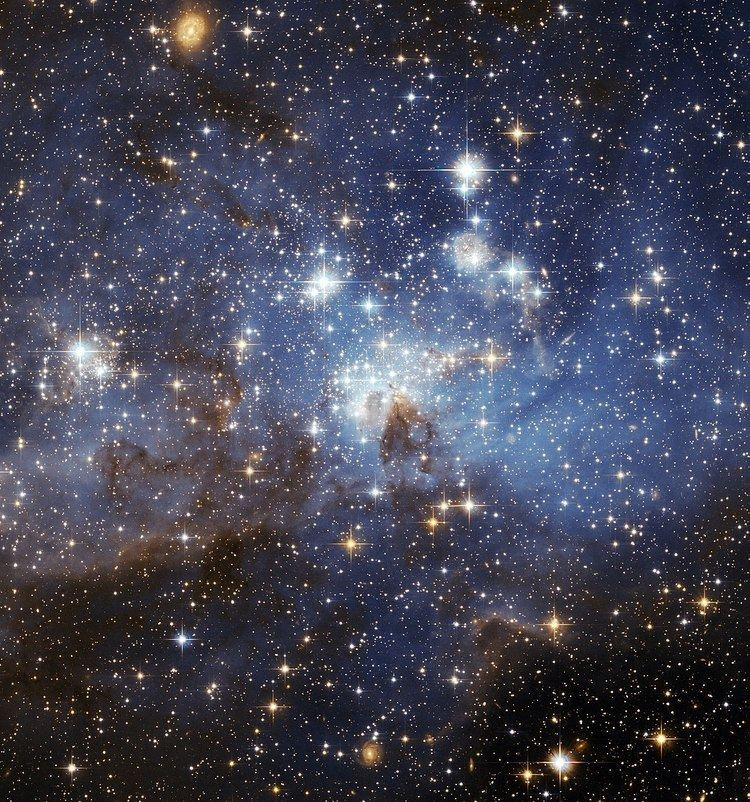# Initial mass function

Updated on
Covid-19In astronomy, the initial mass function (IMF) is an empirical function that describes the distribution of initial masses for a population of stars. The IMF is often given as a probability distribution function (PDF) for the mass at which a star enters the main sequence (begins hydrogen fusion). The distribution function can then be used to construct the mass distribution (the histogram of stellar masses) of a population of stars. The properties and evolution of a star are closely related to its mass, so the IMF is an important diagnostic tool for astronomers studying large quantities of stars. For example, the initial mass of a star is the primary factor determining its colour, luminosity, and lifetime. The IMF is relatively invariant from one group of stars to another, though some observations suggest that the IMF is different in different environments.

## Form of the IMF

The IMF is often stated in terms of a series of power laws, where N ( m ) d m (sometimes also represented as ξ ( m ) Δ m ), the number of stars with masses in the range m to m + d m within a specified volume of space, is proportional to m α , where α is a dimensionless exponent. The IMF can be inferred from the present day stellar luminosity function by using the stellar mass-luminosity relation together with a model of how the star formation rate varies with time. Commonly used forms of the IMF are the Kroupa (2001) broken power law and the Chabrier (2003) log-normal.

## Salpeter (1955)

The IMF of stars more massive than our sun was first quantified by Edwin Salpeter in 1955. His work favoured an exponent of α = 2.35 . This form of the IMF is called the Salpeter function or a Salpeter IMF. It shows that the number of stars in each mass range decreases rapidly with increasing mass. The Salpeter Initial Mass Function is

ξ ( m ) Δ m = ξ 0 ( m M s u n ) 2.35 ( Δ m M s u n ) .

## Miller-Scalo (1979)

Later authors extended the work below one solar mass (M). Glenn E. Miller and John M. Scalo suggested that the IMF "flattened" (approached α = 1 ) below one solar mass.

## Chabrier (2003)

Chabrier 2003 for individual stars:

ξ ( m ) Δ m = 0.158 ( 1 / ( ln ( 10 ) m ) ) exp [ ( log ( m ) log ( 0.08 ) ) 2 / ( 2 × 0.69 2 ) ] for m < 1 , ξ ( m ) = k m α for m > 1 , α = 2.3 ± 0.3

Chabrier 2003 for stellar systems (e.g. binaries):

ξ ( m ) Δ m = 0.086 ( 1 / ( ln ( 10 ) m ) ) exp [ ( log ( m ) log ( 0.22 ) ) 2 / ( 2 × 0.57 2 ) ] for m < 1 , ξ ( m ) = k m α for m > 1 , α = 2.3 ± 0.3

## Kroupa (2001)

Pavel Kroupa kept α = 2.3 above half a solar mass, but introduced α = 1.3 between 0.08-0.5 M and α = 0.3 below 0.08 M.

ξ ( m ) = m α , α = 0.3 for m < 0.08 , α = 1.3 for 0.08 < m < 0.5 , α = 2.3 for 0.5 < m

## Uncertainties

There are large uncertainties concerning the substellar region. In particular, the classical assumption of a single IMF covering the whole substellar and stellar mass range is being questioned in favour of a two-component IMF to account for possible different formation modes of substellar objects. I.e. one IMF covering brown dwarfs and very-low-mass stars on the one hand, and another ranging from the higher-mass brown dwarfs to the most massive stars on the other. Note that this leads to an overlap region between about 0.05 and 0.2 M where both formation modes may account for bodies in this mass range.

## References

Similar Topics
Marc Baumgartner
Peter Gandy (author)
Jakub Hottek
Topics